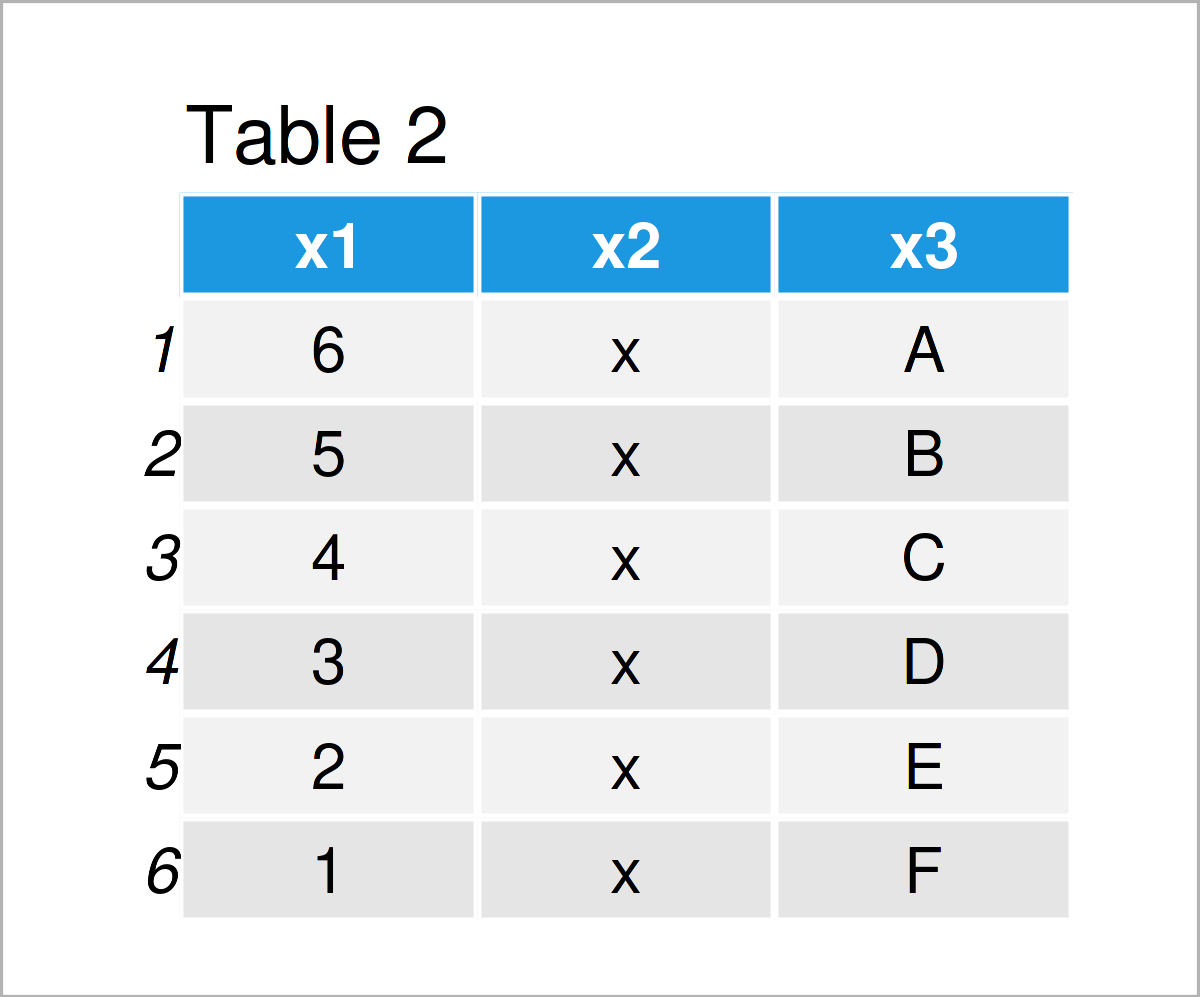# Create Copy of Data Frame in R (2 Examples) | Same & Different Memory Address

In this tutorial, I’ll show how to create a copy of a data frame object in R programming.

The page looks as follows:

## Introducing Example Data

The first step is to create some example data:

```data <- data.frame(x1 = 6:1,              # Create example data
x2 = "x",
x3 = LETTERS[1:6])
data                                      # Print example data```As you can see based on Table 1, the example data is a data frame containing six rows and three columns. The column x1 is an integer and the columns x2 and x3 have the character class.

## Example 1: Create Copy of Data with Same Memory Address

In Example 1, I’ll show how to construct a duplicate of our data frame with the same memory address.

To accomplish this, we can simply use an assignment arrow as shown below:

```data_copy1 <- data                        # Create copy of data
data_copy1                                # Print copy of data```After executing the previous R code the data frame shown in Table 2 has been created. As you can see, this data frame contains exactly the same values as our input data frame.

We can now use the tracemem function to test whether both of our data frames have the same memory address:

```tracemem(data) == tracemem(data_copy1)    # Check if memory addresses are the same
#  TRUE```

The previous R code has returned the logical indicator TRUE, i.e. both data sets have the same memory address.

## Example 2: Create Copy of Data with Different Memory Address

Example 2 illustrates how to replicate a data frame with a different memory address.

For this task, we have to wrap the data.frame function around our input data set:

```data_copy2 <- data.frame(data)            # Create copy of data
data_copy2                                # Print copy of data```In Table 3 it is shown that we have created another version of our data frame using the previous R programming syntax.

Let’s test if the memory address is still identical:

```tracemem(data) == tracemem(data_copy2)    # Check if memory addresses are the same
#  FALSE```

The RStudio console returns the logical value FALSE, i.e. the two data sets do not have the same memory address.

## Video & Further Resources

If you need more explanations on the contents of this page, you might watch the following video on my YouTube channel. I explain the R syntax of this article in the video:

Please accept YouTube cookies to play this video. By accepting you will be accessing content from YouTube, a service provided by an external third party.If you accept this notice, your choice will be saved and the page will refresh.

Additionally, you might have a look at some of the related RStudio tutorials on this website. I have published several articles already:

Summary: This article has demonstrated how to construct a copy of a data frame object in R. If you have further questions, don’t hesitate to let me know in the comments below.

Subscribe to the Statistics Globe Newsletter